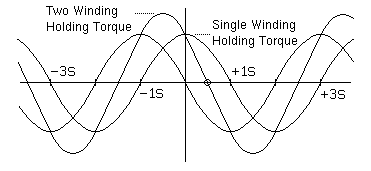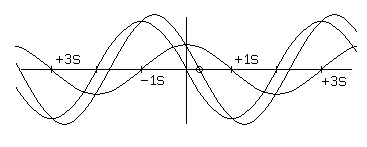Electronic Circuits and Tutorials
Tutorials
Dictionaries
Projects
Facebook
Discover
Home > Electronics Tutorial > Stepper Motor Physics > Half Stepping and Micro Stepping
STEPPING MOTOR PHYSICS TUTORIALS

# Half Stepping and Micro Stepping

So long as no part of the magnetic circuit saturates, powering two motor windings simultaneously will produce a torque versus position curve that is the sum of the torque versus position curves for the two motor windings taken in isolation. For a two-winding permanent magnet or hybrid motor, the two curves will be S radians out of phase, and if the currents in the two windings are equal, the peaks and valleys of the sum will be displaced S/2 radians from the peaks of the original curves, as shown in Figure 2.2:
```Figure 2.2```

This is the basis of half-stepping. The two-winding holding torque is the peak of the composite torque curve when two windings are carrying their maximum rated current. For common two-winding permanent magnet or hybrid stepping motors, the two-winding holding torque will be:

h2 = 20.5 h1
where:
h1 -- single-winding holding torque
h2 -- two-winding holding torque
This assumes that no part of the magnetic circuit is saturated and that the torque versus position curve for each winding is an ideal sinusoid.

Most permanent-magnet and variable-reluctance stepping motor data sheets quote the two-winding holding torque and not the single-winding figure; in part, this is because it is larger, and in part, it is because the most common full-step controllers always apply power to two windings at once.

If any part of the motor's magnetic circuits is saturated, the two torque curves will not add linearly. As a result, the composite torque will be less than the sum of the component torques and the equilibrium position of the composite may not be exactly S/2 radians from the equilibria of the original.

Microstepping allows even smaller steps by using different currents through the two motor windings, as shown in Figure 2.3:

```Figure 2.3```

For a two-winding variable reluctance or permanent magnet motor, assuming no saturating magnetic circuits, and assuming perfectly sinusoidal torque versus position curves for each motor winding, the following formula gives the key characteristics of the composite torque curve:

h = ( a2 + b2 )0.5
x = ( S / ( /2) ) arctan( b / a )
Where:
a -- torque applied by winding with equilibrium at 0 radians.
b -- torque applied by winding with equilibrium at S radians.
h -- holding torque of composite.
x -- equilibrium position, in radians.
S -- step angle, in radians.
In the absence of saturation, the torques a and b are directly proportional to the currents through the corresponding windings. It is quite common to work with normalized currents and torques, so that the single-winding holding torque or the maximum current allowed in one motor winding is 1.0.

Note: To report broken links or to submit your projects, tutorials please email to Webmaster